Homework Help Question & Answers

# A horizontal compass is placed 17 cm due south from a straight vertical wire carrying a...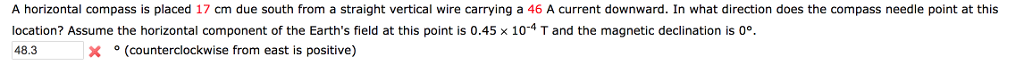A horizontal compass is placed 17 cm due south from a straight vertical wire carrying a 46 A current downward. In what direction does the compass needle point at this location? Assume the horizontal component of the Earth's field at this point is 0.45 x 10-4 T and the magnetic declination is 00. 48. X。(counterclockwise from east is positive)

#### Homework Answers

Answer #1

B = mu0 I / 2 pi r

B = (4 pi * 10-7 * 46) / (2 pi * 0.17) = 5.41 * 10-5 T

Bnet = sqrt [(5.41 * 10-5)2 + (0.45 * 10-4)2]

Bnet = 7.04 * 10-5 T

theta = tan-1 [0.45 * 10-4 / 5.41 * 10-5]

theta = 39.7 deg

Know the answer?
Your Answer:

#### Post as a guest

Your Name:

What's your source?

#### Earn Coin

Coins can be redeemed for fabulous gifts.

Not the answer you're looking for? Ask your own homework help question. Our experts will answer your question WITHIN MINUTES for Free.
Similar Homework Help Questions
• ### A horizontal compass is placed 21 cm due south from a straight vertical wire carrying a...

A horizontal compass is placed 21 cm due south from a straight vertical wire carrying a 35 A current downward. In what direction does the compass needle point at this location? Assume the horizontal component of the Earth's field at this point is 0.45 ✕ 10-4 T and the magnetic declination is 0°. Calculate degree and specify direction.

• ### Problem 5 Compass S A horizontal compass is placed 0.100 em due South from a straight...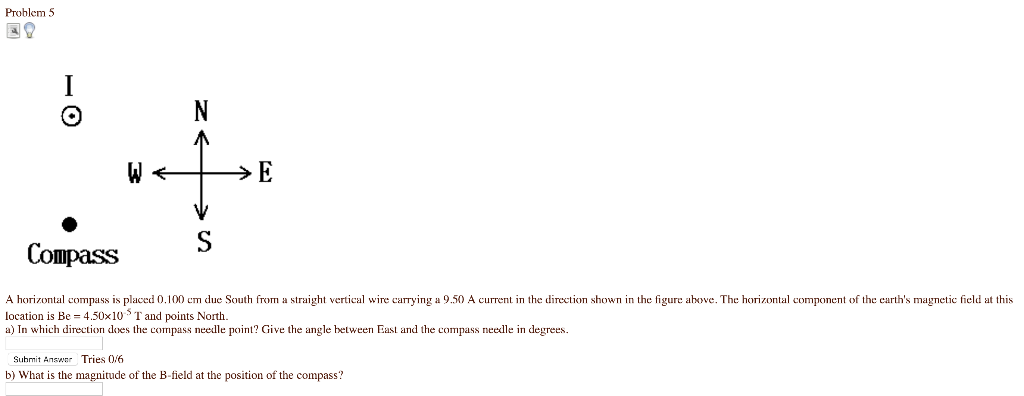Problem 5 Compass S A horizontal compass is placed 0.100 em due South from a straight vertical wire carrying a 9.50 A current in the direction shown in the figure above. The horizontal component of the earth's magnetic field at this location is Be 450x10 T and points North a) In which direction does the compass needle point? Give the angle between Easl and the compass needle in degrees Suhmit Anwr Tries 0/6 b) What is the magnitude of the...

• ### A long,straight current-carrying wire runs from north to south

A long,straight current-carrying wire runs from north to south. A) A compass needle placed above the wire points with its N-pole toward the east. In what direction is the current flowing? B) If a compass is put underneath the wire, which direction will the compass neddle point?

• ### A long current-carrying wire, oriented North-South, lies on a table (it is connected to batteries which...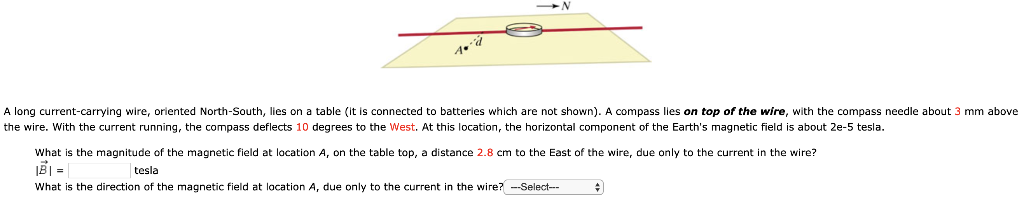A long current-carrying wire, oriented North-South, lies on a table (it is connected to batteries which are not shown). A compass lies on top of the wire, with the compass needle about 3 mm above the wire. With the current running, the compass deflects 10 degrees to the West. At this location, the horizontal component of the Earth's magnetic field is about 2e-5 tesla. What is the magnitude of the magnetic field at location A, on the table top, a...

• ### nt FULL SCREEN PRINTER VERSION NEXT Cnapter 41, ProDiem b2 A smail compass is held horizontally,...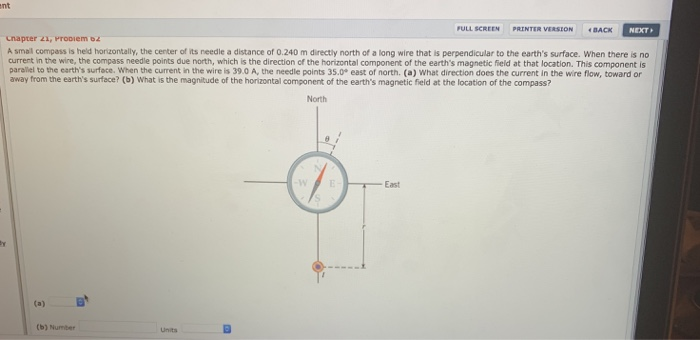nt FULL SCREEN PRINTER VERSION NEXT Cnapter 41, ProDiem b2 A smail compass is held horizontally, the center of its needle a distance of 0.240 m directly north of a long wire that is perpendicular to the earth's surface. When there is no current in the wire, the compass needle points due north, which is the direction of the horizontal component of the earth's magnetic field at that location. This component is paraliel to the earth's surface. When the current...

• ### A long, straight wire carrying a current I1 is placed on a horizontal table in front...

A long, straight wire carrying a current I1 is placed on a horizontal table in front of you and the direction of the current points +x axis. The magnetic field produced by the current I1 at a point 5 cm above the wire is 0.2 T. A second parallel wire carrying a current I2 = 3I1 is placed 10 cm above the first wire and the direction of the current also points +x axis. What is the direction of the...

• ### 2. A very long, straight wire runs from west to east. The wire is 17 m...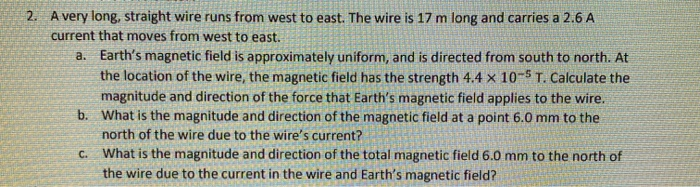2. A very long, straight wire runs from west to east. The wire is 17 m long and carries a 2.6 A current that moves from west to east. a. Earth's magnetic field is approximately uniform, and is directed from south to north. At the location of the wire, the magnetic field has the strength 4.4 x 10-5 T. Calculate the magnitude and direction of the force that Earth's magnetic field applies to the wire. b. What is the magnitude...

• ### A long, straight, north-south wire is carrying a current northward of 15 A. a) What is...

A long, straight, north-south wire is carrying a current northward of 15 A. a) What is the magnitude and direction of the magnetic field at a location 0.4 m directly to the east of this wire? b) If a proton at the location in Part A was traveling away from the wire at a velocity of 8,000 km/s, calculate the magnitude and justify the direction of the magnetic force on this proton?

• ### hw5 6 A wire is connected to batteries (not shown), aA wire is connected to batteries (not shown), and current flows through the wire. The wire lies flat on a table, and you are looking down on it from above. Thewire is laid on top of a compass, resting about 3 mm above the needle. The distance d is 5.5 cm. The compass needle deflects18 degrees from North, as shown. At this location the horizontal component of theEarth's magnetic field is 2e-5 tesla.What is the direction of the magnetic field...

• ### In Oersted's experiment, suppose that the compass was 0.25 m from the current-carrying wire. If a...

In Oersted's experiment, suppose that the compass was 0.25 m from the current-carrying wire. If a magnetic field of half the Earth's magnetic field of 5.0×10−5T was required to give a noticeable deflection of the compass needle, what current must the wire have carried?